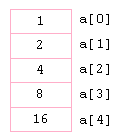Array sort - C# , VB.NetArrays are using for store similar data types grouping as a single unit. Instead of declaring individual variables, such as arr1, arr2, arr3 etc. you declare one array variable such as numbers and use arr, arr, arr etc. to represent individual variables. A specific element in an array is accessed by an index.

Integer Array Example

C#

```int[] array = new int;
array = 10;
array = 20;
array = 30;
array = 40;
```

VB.Net

```Dim array As Integer() = New Integer(3) {}
array(0) = 10
array(1) = 20
array(2) = 30
array(3) = 40
```

String Array Example

C#

```string[] week = new string;
week = "Sunday";
week = "Monday";
.....
```

VB.Net

```Dim week(6) As String
week(0) = "Sunday"
week(1) = "Monday"
week(2) = "Tuesday"
.....
```

Sorting ArraysYou can sort the arrays in ascending order as well as descending . We can use Array.Sort method for sorts the elements in a one-dimensional array.

How to sort an Integer Array

The following source code shows how to sort an integer Array in ascending order.

C#

```int[] array = new int[] { 3, 1, 4, 5, 2 };
Array.Sort(array);
foreach (var str in array)
{
MessageBox.Show(str.ToString());
}
```

VB.Net

```Dim arr As Integer() = New Integer() {3, 1, 4, 5, 2}
Array.Sort(arr)
For Each str As Integer In arr
MsgBox(str)
Next
```

How to sort a String Array

The following code shows how to sort a string Array in ascending orde.

C#

```string[] ArrStr = new string[] { "c", "a", "d", "b" };
Array.Sort(ArrStr);
foreach (var str in ArrStr)
{
MessageBox.Show(str);
}
```

VB.Net

```Dim ArrStr As String() = New String() {"c", "a", "d", "b"}
Array.Sort(ArrStr)
For Each str As String In ArrStr
MsgBox(str)
Next
```

Sort Array in descending orderAbove code shows how to sort an Array in ascending order, like that, you can sort an Array in descending order. We can use Array.Reverse method for reverses the sequence of the elements in the entire one-dimensional Array.

Sort an Integer array in descending order

The following code shows how to sort an Integer array in reverse order.

C#

```int[] array = new int[] { 3, 1, 4, 5, 2 };
Array.Sort(array);
Array.Reverse(array);
foreach (var str in array)
{
MessageBox.Show(str.ToString());
}
```

VB.Net

```Dim arr As Integer() = New Integer() {3, 1, 4, 5, 2}
Array.Sort(arr)
Array.Reverse(arr)
For Each str As Integer In arr
MsgBox(str)
Next
```

Sort a String array in descending order

The following code shows how to sort a string array in reverse order.

C#

```string[] array = new string[] { "c", "b", "d", "a" };
Array.Sort(array);
Array.Reverse(array);
foreach (var str in array)
{
MessageBox.Show(str.ToString());
}
```

VB.Net

```Dim ArrStr As String() = New String() {"c", "a", "d", "b"}
Array.Sort(ArrStr)
Array.Reverse(ArrStr)
For Each str As String In ArrStr
MsgBox(str)
Next
```

LINQ OrderByDescending

You can use LINQ OrderByDescending method to reverse an array. OrderByDescending method sorts elements from high to low. The following C# source code shows how to sort an array in descending order by using LINQ OrderByDescending.

```string[] array = new string[] { "a", "b", "c", "d" };
array = array.OrderByDescending(c => c).ToArray();
foreach (var str in array)
{
MessageBox.Show(str.ToString());
}
```

Array Example C#, VB.Net

Arrays are using for store similar data types grouping as a single unit. We can access Array elements by its numeric index. The array indexes start at zero. More about.... C# Array , VB.Net Array

How to Create an Array with different data types

You can create an array with elements of different data types when declare the array as Object. Since System.Object is the base class of all other types, an item in an array of Objects can have a reference to any other type of object. More about.... Multiple data types in an Array

NEXT.....Image to Byte Array